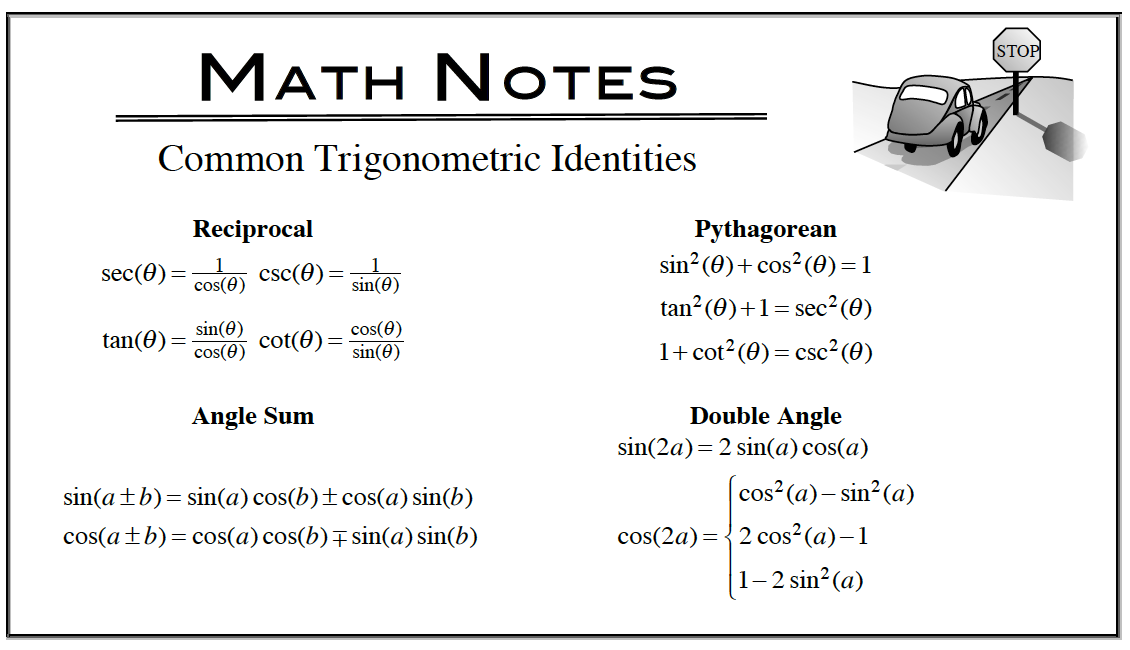### Home > APCALC > Chapter 8 > Lesson 8.1.1 > Problem8-8

8-8.

No calculator! Evaluate the following integrals. Homework Help ✎

1. $\int \frac { d } { d x } ( e ^ { 3 x } \operatorname { sin } ( 5 x ) ) d x$

Remember that the integral 'undoes' the derivative.But notice that you are integrating as a final step: don't forget $+$$C$.

1. $\int _ { 3 } ^ { 5 } e ^ { 4 x + 2 } d x$Recall that $e^{4x + 2} = e^2e^{4x}$ and $e^2$ is a constant.

1. $\int \frac { \operatorname { sec } ( x ) \operatorname { sin } ( x ) } { \operatorname { cos } ( x ) } d x$

$\text{Recall that sec}(x)=\frac{1}{\text{cos}(x)}\text{ and }\text{tan}(x)=\frac{\text{sin}(x)}{\text{cos}(x)}?$

Before integrating, rewrite the integrand so it is not a fraction.

1. $\int \operatorname { sin } ( 2 x ) \cdot 2 ^ { \operatorname { sin } ^ { 2 } ( x ) } d x$

Use $u$-substitution.
$u = \sin^2(x)$1. $\int _ { 0 } ^ { 2 } x ( 4 x - 2 ) ^ { 2 } d x$

Before integrating, expand the integrand.

$=\int_0^2\Big(16x^3-16x^2+4x\Big)dx$